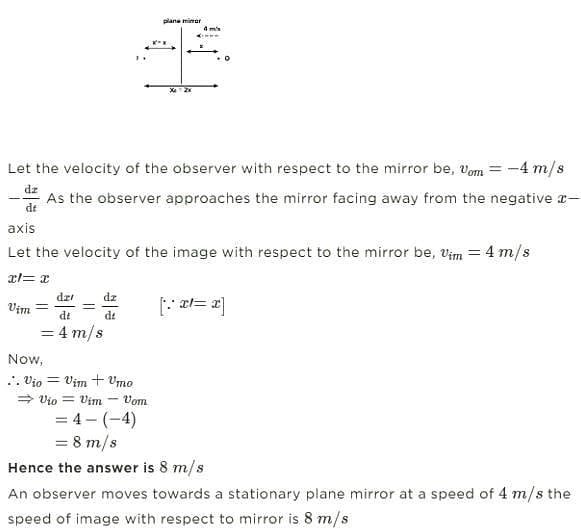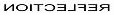Test: Reflection of Light

# Test: Reflection of Light - Class 10

Test Description

## 20 Questions MCQ Test Science Class 10 - Test: Reflection of Light

Test: Reflection of Light for Class 10 2023 is part of Science Class 10 preparation. The Test: Reflection of Light questions and answers have been prepared according to the Class 10 exam syllabus.The Test: Reflection of Light MCQs are made for Class 10 2023 Exam. Find important definitions, questions, notes, meanings, examples, exercises, MCQs and online tests for Test: Reflection of Light below.
Solutions of Test: Reflection of Light questions in English are available as part of our Science Class 10 for Class 10 & Test: Reflection of Light solutions in Hindi for Science Class 10 course. Download more important topics, notes, lectures and mock test series for Class 10 Exam by signing up for free. Attempt Test: Reflection of Light | 20 questions in 20 minutes | Mock test for Class 10 preparation | Free important questions MCQ to study Science Class 10 for Class 10 Exam | Download free PDF with solutions
 1 Crore+ students have signed up on EduRev. Have you?
Test: Reflection of Light - Question 1

### Raj is standing in front of a plane mirror. The distance between Raj and his image is 100cm. If the distance between the mirror and image is x. What could be the possible value of x ?

Detailed Solution for Test: Reflection of Light - Question 1

Distance between raj and image=100cm
Distance between image mirror =x
Distance between image and mirror= Distance between mirror and raj
x+x=100
2x=100
x=50cm

Test: Reflection of Light - Question 2

### Virtual image forms

Detailed Solution for Test: Reflection of Light - Question 2

A virtual image occurs when light rays do not actually meet at the image. Instead, you " See" the image because your eye projects light rays backward. In flat, or plane mirrors, the image isa virtual image, and is the same distance behind the mirror as the object is in front of the mirror.

Test: Reflection of Light - Question 3

### Which among the following is not true for a plane mirror ?

Detailed Solution for Test: Reflection of Light - Question 3

The image formed by a plane mirror is always virtual,upright, and of the same shape and size as the object it is reflecting. A virtual image is a copy of an object formed at the location from which the light rays appear to come.

Test: Reflection of Light - Question 4

The focal length of a plane mirror is

Detailed Solution for Test: Reflection of Light - Question 4

The focal length of a plane mirror is infinity as the image can be formed at an infinite distance inside the mirror.

Test: Reflection of Light - Question 5

The image formed by plane mirror is

Detailed Solution for Test: Reflection of Light - Question 5

The image formed by a plane mirror is always virtual (meaning that the light rays do not actually come from the image), upright, and of the same shape and size as the object it is reflecting. A virtual image is a copy of an object formed at the location from which the light rays appear to come.

Test: Reflection of Light - Question 6

An observer moves towards a stationary plane mirror at a speed of 4m/s. The speed of image with respect to mirror is __________.

Detailed Solution for Test: Reflection of Light - Question 6

Step 1: Given data

An observer moves towards a stationary plane mirror at a speed of 4m/s

Step 2: Find the speed of the image with respect to the mirror

The mirror and the object move toward each other As a result, there is a relative velocity between the object and the mirror. Similarly, the image will move toward the mirror in a manner that is comparable to that of the object. The relative velocity of the image in relation to the mirror allows us to calculate its relative velocity in relation to the ground.Test: Reflection of Light - Question 7is the lateral inversion of

Detailed Solution for Test: Reflection of Light - Question 7

Lateral inversion is a phenomenon in which left appears to be right and vice versa. It is due to direction that light follows when it strikes a reflecting surface, generally a mirror.

Test: Reflection of Light - Question 8

Which of the following letter does not show lateral inversion ?

Detailed Solution for Test: Reflection of Light - Question 8

Lateral version of I is I

Test: Reflection of Light - Question 9

A medium through which light propagates easily is a

Detailed Solution for Test: Reflection of Light - Question 9

In the field of optics, transparency (also called pellucidity or diaphaneity) is the physical property of allowing light to pass through the material without being scattered.

Test: Reflection of Light - Question 10

Virtual image

Detailed Solution for Test: Reflection of Light - Question 10

A virtual image is defined as the opposite of a real image, therefore an image that cannot be obtained on a screen is reffered to as a virtual image. The rays of light that form a virtual image never converge therefore a virtual image can never be projected onto a screen.

Test: Reflection of Light - Question 11

Real image

Detailed Solution for Test: Reflection of Light - Question 11

A real image occurs where rays converge, whereas a virtual image occurs where rays only appear to converge. Real images can be produced by concave mirrors and converging lenses, only if the object is placed further away from the mirror/lens than the focal point and this real image is inverted.

Test: Reflection of Light - Question 12

What will be the angle of reflection if light falls normally on the reflecting surface?

Detailed Solution for Test: Reflection of Light - Question 12

For a plane mirror: If the incident ray falls along the normal, the angle of incidence is 0 degrees, NOT 90 degrees. The angle is measured with the normal to the mirror and not with the plane of the mirror. Hence the angle of reflection is equal to 0 degrees.

Test: Reflection of Light - Question 13

Angle of incidence is

Detailed Solution for Test: Reflection of Light - Question 13

Angle of incidence is the angle which an incident line or ray makes with a perpendicular to the surface at the point of incidence.

Test: Reflection of Light - Question 14

Angle of reflection is

Detailed Solution for Test: Reflection of Light - Question 14

Angle of reflection is the angle between ghe reflected ray and the normal angle of incidence is the angle between the incident ray and the normal always angle of incidence =angle of reflection

Test: Reflection of Light - Question 15

Which of the following letter shows lateral inversion ?

Detailed Solution for Test: Reflection of Light - Question 15

Letter L shows lateral inversion

Test: Reflection of Light - Question 16

Rahul is standing in front of a plane mirror. The distance between Rahul and his image is 150cm. If Rahul’s distance from the mirror is x. What could be the possible value of x ?

Detailed Solution for Test: Reflection of Light - Question 16

x = 75 cm, as for a plane mirror the distance between the object and the mirror is equal to the distance between the mirror and image.

Test: Reflection of Light - Question 17

Light always travels along a

Detailed Solution for Test: Reflection of Light - Question 17

Light follows rectilinear motion. It travels in straight line. A light wave can be considered to travel from one point to another along a straight line joining them.

Test: Reflection of Light - Question 18

Harry is standing in front of a plane mirror at a distance of 6 m. His image on the other side will form at a distance of

Detailed Solution for Test: Reflection of Light - Question 18

For reflection through a plane mirror, Image distance (v) = Object distance (u) = 6 m

Test: Reflection of Light - Question 19

In a lateral inversion

Detailed Solution for Test: Reflection of Light - Question 19

The left and right of a mirror image appear reversed ( lateral inversion) because of how we perceive the mirror image. The phenomenon where your left appears as the right and vice versa in a mirror is reffered to as lateral inversion.

Test: Reflection of Light - Question 20

Which among the following is true for a plane mirror ?

Detailed Solution for Test: Reflection of Light - Question 20

The normal line divides the angle between the incident ray and the reflected ray into two equal angles. The angle between the incident ray and the normal is known as the angle of incidence. The angle between the reflected ray and the normal is known as the angle of reflection.

## Science Class 10

78 videos|509 docs|153 tests
Information about Test: Reflection of Light Page
In this test you can find the Exam questions for Test: Reflection of Light solved & explained in the simplest way possible. Besides giving Questions and answers for Test: Reflection of Light, EduRev gives you an ample number of Online tests for practice

## Science Class 10

78 videos|509 docs|153 tests